# POD and DMD Reduced Order Models for a 2D Burgers Equation

Authors: - 07 March 2020

# Burgers equation

For a given viscosity parameter $\nu$ and for time $t>0$, we consider the 2D Burgers equation on the unit square

with zero Neumann boundary conditions and initial condition

and its numerical approximation using

1. Finite Elements in space and Runge-Kutta in time,
2. a POD reduction of the FEM model, and
3. a DMD reduced order model.

We present the basic ideas and how they are realized in a basic Python script.

# 0. The Setup

Please download and run the python file burgers.py to check the implementation, to reproduce the presented results, and to the behavior of the routines for different parameters.

Here is the start of the script that lists the modules on which the implementation bases on.

import dolfin

import scipy.linalg as spla
import matplotlib.pyplot as plt
import numpy as np

from scipy.integrate import solve_ivp
from spacetime_galerkin_pod.ldfnp_ext_cholmod import SparseFactorMassmat


The code uses dolfin which is the python interface to FEniCS while the other modules scipy, numpy, and matplotlib are standard in python, I would say. The module ldfnp_ext_cholmod is a little wrapper for the sparsity optimizing Cholesky decomposition of sksparse. It is available from my github repository and falls back to numpy routines, in the case that sksparse is not available.

# 1. The FEM Discretization

## The spatial discretization in FEniCS

First, the mesh is defined – here a uniform triangulation of the unit square controlled by the parameter N. For the value N=80 and for globally smooth and piecewise quadratic ansatz functions, the resulting dimension of the system is 51842.

# The mesh

pone = dolfin.Point(-1, -1)
ptwo = dolfin.Point(1, 1)
mesh = dolfin.RectangleMesh(pone, ptwo, N, N)

V = dolfin.VectorFunctionSpace(mesh, 'CG', 2)

### The FENICS FEM Discretization

v = dolfin.TestFunction(V)
u = dolfin.TrialFunction(V)


Next, the discrete linear operators are defined and exported as a SciPy sparse matrix. Also, we factorize the mass matrix mmat for later use and define the norm that is weighted with the mass matrix as this is the discrete $L^2$ norm.

# ## the mass matrix

mform = dolfin.inner(v, u)*dolfin.dx
massm = dolfin.assemble(mform)
mmat = dolfin.as_backend_type(massm).sparray()
mmat.eliminate_zeros()

# factorize it for later

mfac = SparseFactorMassmat(mmat)

# norm induced by the mass matrix == discrete L2-norm

def mnorm(uvec):
return np.sqrt(np.inner(uvec, mmat.dot(uvec)))

# ## the stiffness matrix
# as a form in FEniCS

aassm = dolfin.assemble(aform)

# as a sparse matrix

amat = dolfin.as_backend_type(aassm).sparray()
amat.eliminate_zeros()


Then we define the convective term as function of the velocity u both in terms of an FE-function and as a function of the associated coefficient vector.

# ## the convective term

# as a function in FEniCS

def burgers_nonl_func(ufun):
cass = dolfin.assemble(cform)
return cass

# as a vector to form map

def burgers_nonl_vec(uvec):
ufun = dolfin.Function(V)
ufun.vector().set_local(uvec)
bnlform = burgers_nonl_func(ufun)
bnlvec = bnlform.get_local()
return bnlvec


## The Runge-Kutta Time Integration

To apply standard time integration schemes (here, RK23 turned out to be most efficient), we define the right hand side $f_h$ of the spatially discretized problem

In this case the right hand side is an application of the discrete diffusion and convection operator and the inverse of the mass matrix that, simply speaking, maps a (discrete) form onto a discrete function. Note that there is no explicit time dependency in the Burgers equation, but SciPy’s solve_ivp requires this parameter. Also, we define the initial value here. The time grid is used to store the solution for the snapshots needed later, but the time integrator uses his internal time grid.

inivstrg = 'exp(-3.*(x*x+x*x))'
inivexpr = dolfin.Expression((inivstrg, inivstrg), degree=2)
inivfunc = dolfin.interpolate(inivexpr, V)
inivvec = inivfunc.vector().get_local()

burgsol = solve_ivp(brhs, (t0, tE), inivvec, t_eval=timegrid, method='RK23')
fullsol = burgsol.y


Here is the result. Note the sharp front that develops towards the end of the time integration.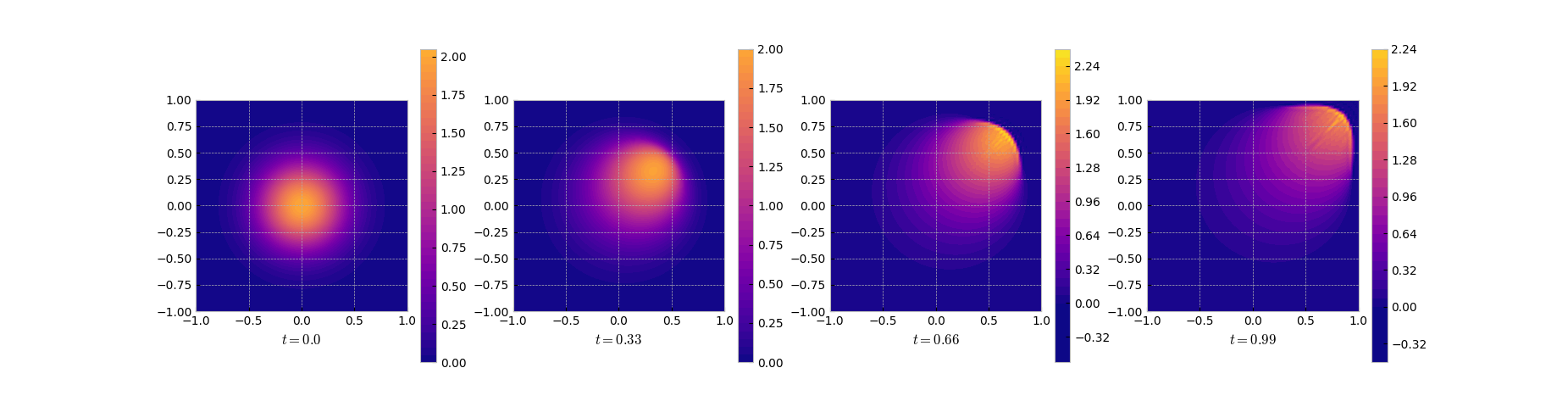Solution snapshots of the full FEM model

# 2. POD Reduced Model

If one has snapshots of the solution $v _ h$ at some time instances $t _ i$ one may well think that the span of the matrix of snapshots

is a good candidate for a space in which the solution evolves in. One may even go further and look for a low-dimensional basis of this space. The span of a matrix is best approximated by its dominant singular vectors. And this is the idea of Proper Orthogonal Decomposition (POD) – use the leading singular vectors as a basis for the solution space.

We use the Nts=101 snapshots of the FEM solutions to setup the matrix of measurements $X$ and to compute the POD modes as $M^{-1/2}v _ k$, where $v _ k$ is the $k$-th leading left singular vector of $M^{1/2}X$. This procedure gives a low-dimensional orthogonal (in the discrete $L^2$ inner product) basis that optimally parametrizes the subspace of $L^2$ that is spanned by the solution snapshots1. In this example, we use the poddim=25 leading singular vectors to define the reduced model.

snapshotmat = mfac.Ft.dot(burgsol.y)
podmodes, svals, _ = spla.svd(snapshotmat, full_matrices=False)
selected_podmodes = podmodes[:, :poddim]
podvecs = mfac.solve_Ft(selected_podmodes)


In this implementation we use a sparse factor of the mass matrix instead of the square root. The singular values (in particular those that correspond to the discarded directions) give an indication of how good the approximation is.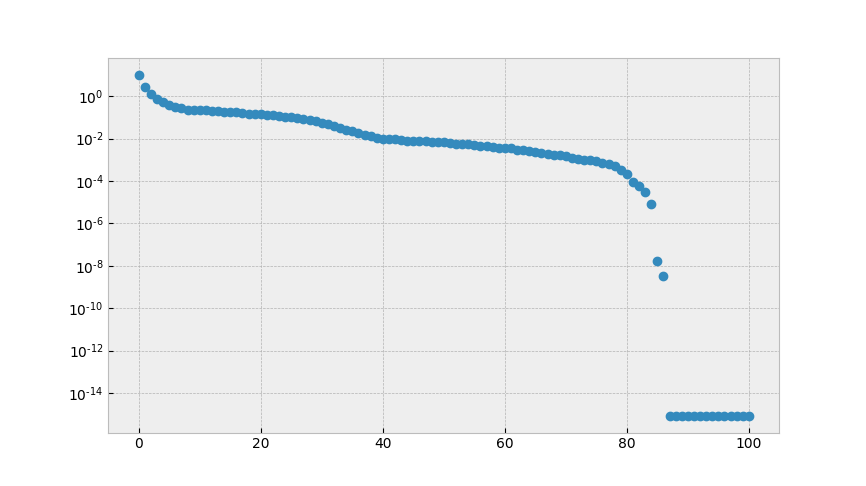The singular values of the snapshot matrix

Here, the decay is comparatively slow, so that a one should not expect a good low dimensional approximation by POD.

For the simulation, the state is parametrized by $u_h (t) \approx V \tilde u_h(t)$ where $V$ is the matrix of the POD modes (in the code $V$ denoted by podvecs), which gives a system in $\tilde u _ h$ with 25 degrees of freedom (as opposed to the 51842 of the full order model).

redamat = podvecs.T.dot(amat.dot(podvecs))  # the projected stiffness

def redbrhs(time, redvec):
inflatedv = podvecs.dot(redvec)
redconv = podvecs.T.dot(burgers_nonl_vec(inflatedv))
return -redamat.dot(redvec) - redconv.flatten()


Here we define the projected stiffness matrix and the reduced nonlinearity through

1. inflating the reduced state to full dimension
2. applying the nonlinearity
3. projecting down the result.

This means that our model is not completely independent of the full dimension. For this problem there are hyperreduction techiques like DEIM.

Thus, the right hand side is readily defined the more that the projected mass matrix is the identity. Why?

Finally, the initial value is projected into the reduced coordinates and the reduced system is integrated in time.

redburgsol = solve_ivp(redbrhs, (t0, tE), prjinivvec,
t_eval=timegrid, method='RK23')
podredsol = redburgsol.y


In the solution we see that the reduced order model gives a decent approximation in the smooth regime in the beginning and has its troubles approximating the front as can be seen in the error (log) plot.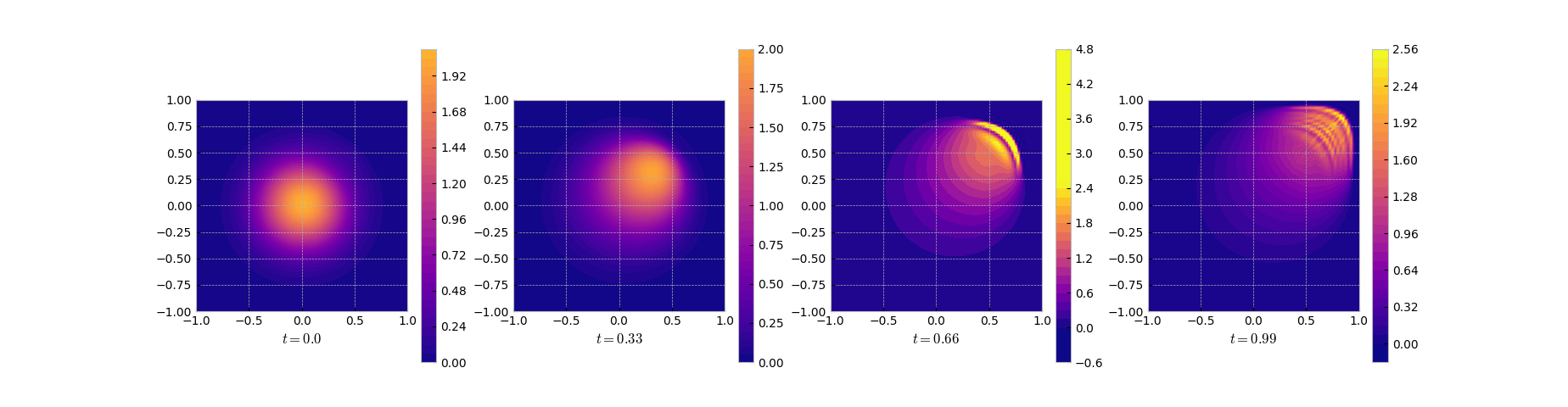Snapshots of the solution of the reduced system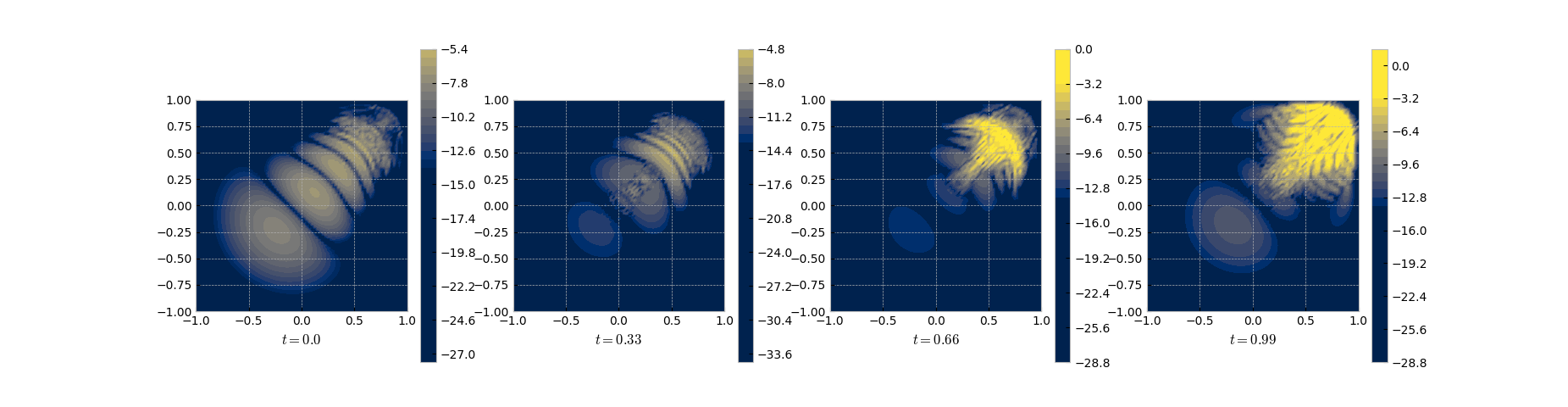Snapshots of the log of the error between the full and the POD solution

# 3. DMD Reduced Model

POD is partially data driven – it uses data to create a basis but still uses (a projection of) the model. If only snapshots but no model is given, one may use the method of Dynamic Mode Decomposition2 (DMD) that tries to identify a matrix $A$ that evolves the state like

In practice, one uses a set of snapshots and the two measurement matrices

and

Note that $X’$ is basically $X$ shifted by one time step.

Then the DMD matrix can be found by solving the linear regression problem

For the reduced DMD model we use the same snapshot matrix as for the POD. The regression problem is solved via SVD to compute the needed pseudo inverse since this naturally allows for a rank reduction and a factored representation of the DMD matrix.

# ### dmd using truncated svd inverse

fburgsol = burgsol.y
Xmat = fburgsol[:, :-1]
Xdsh = fburgsol[:, 1:]
ux, sx, vxh = spla.svd(Xmat, full_matrices=False)
uxr, sxr, vxhr = ux[:, :poddim], sx[:poddim], vxh[:poddim, :]

# compute the dmd matrix in factored form: dmda = dmdaone * dmdatwo

dmdaone = Xdsh.dot(vxhr.T)
dmdatwo = np.linalg.solve(np.diag(sxr), uxr.T)


Once the DMD matrix $A$ is determined, the simulation of the DMD reduced model is only a repeated multiplication by $A$.

# simulation of the dmd reduced model

dmdxo = inivvec
dmdsol = [dmdxo]
for k in np.arange(Nts):
dmdsol.append(dmdaone.dot(dmdatwo.dot(dmdsol[-1])))

dmdsol = np.array(dmdsol).T


As can be seen from the results and the error plots, DMD does a good job in the initial phase but fails in the region with the sharp front.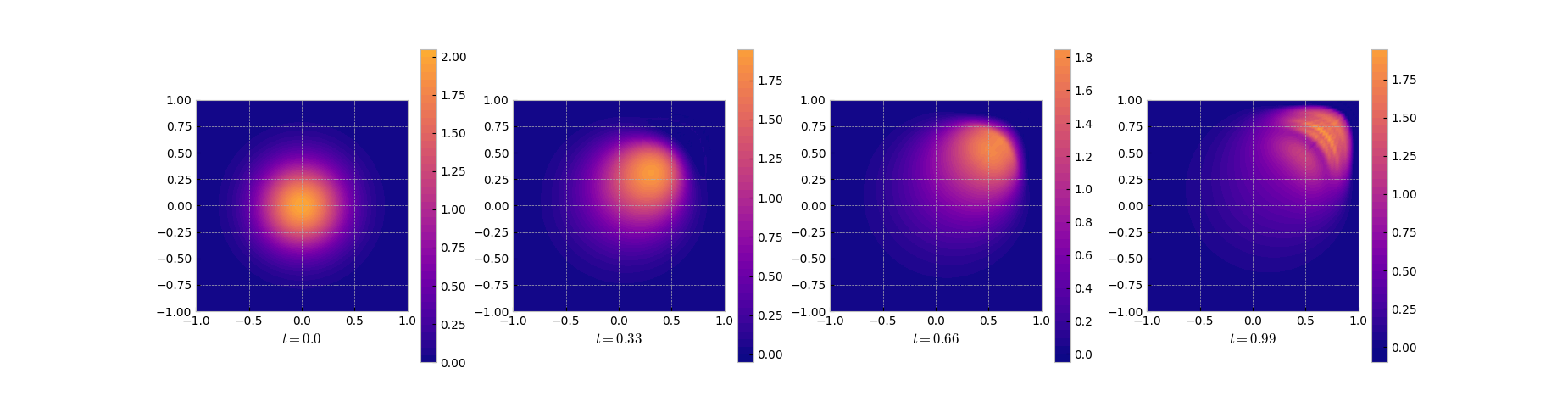Snapshots of the DMD solution

# 4. Remarks

It is commonly accepted that POD does not work well for transport dominated problems – like the current case with the low viscosity parameter nu=1e-4.

So, I think that the results for POD are quite good noting that the reduced order model has 25 degrees of freedom whereas the full model has 51842. Nonetheless, in my tests, increasing the number of basis functions did not help much. One can use a larger nu to get better POD approximations.

The DMD approach shows a similar performance. If compared to POD, the qualitative approximation looks less good but the numbers are slightly better. All in all, the DMD approximation seems less reliable as for some parameter choices, the performance severely deteriorated.

Differential Equations.* arXiv:1611.04050

Decomposition: Theory and Applications* arXiv:1312.0041

1. See, e.g., Lemma 2.5 of Baumann, Benner, and Heiland (2018): *Space-Time Galerkin POD with Application in Optimal Control of Semi-linear Parabolic Partial

2. See, e.g., Tu, Rowley, Luchtenburg, Brunton, Kutz (2013): *On Dynamic Mode